# Analysis of SIF Intrinsic Dynamics

3309 words (13 pages) Essay

12th Mar 2018 Physics Reference this

Tags:

Disclaimer: This work has been submitted by a university student. This is not an example of the work produced by our Essay Writing Service. You can view samples of our professional work here.

Any opinions, findings, conclusions or recommendations expressed in this material are those of the authors and do not necessarily reflect the views of UKEssays.com.

Abstract. It was recently found that spectral solar incident flux (SIF) as a function of the ultraviolet wavelengths exhibit 1/fï€­type power-law correlations. In this study, an attempt was made to explore the SIF intrinsic dynamics versus a wider range of wavelengths, from 115.5nm to 629.5nm. It seemed that the intermittency of SIF data set was very high and the revealed DFA-n exponents were very close to unity. Moreover, the power spectral density was better fitted algebraically than exponentially with exponent very close to unity. Eliminating the fitting of three different types of Planck formula from SIF data set, scaling exponents very close to unity were derived, indicating that the 1/f scaling dynamics concern not the Planck’s law but its variations.

1. Introduction

As it is well known, electromagnetic radiation is continuously emitted by every physical body. This emitted radiation is adequately described by Planck’s law near thermodynamic equilibrium at a definite temperature. There is a positive correlation between the temperature of an emitting body and the Planck radiation at every wavelength. As the temperature of an emitting surface increases, the maximum wavelength of the emitted radiation increases too.

Smith and Gottlieb (1974) re-examined the subject of photon solar flux and its variations versus wavelength and showed that variations in the extreme ultraviolet (UV) spectrum and in the X-ray of solar flux may reach at high orders of magnitude causing significant changes in the Earth’s ionosphere, especially during major solar flares (Kondratyev et al. 1995; Kondratyev and Varotsos 1996; Alexandris et al. 1999; Melnikova 2009; Xue et al. 2011).

Simon (1978) examined the solar irradiance fluxes from 120 to 400 nm and suggested that the internal scaling properties of solar radiation during the eleven-year cycle is still unknown, as the cause of the available solar data discrepancies can not be attributed to solar activity.

Solanki and Unruh (1998) proposed simple models of the total solar irradiance variations versus wavelength showing that variations on solar flux are mainly caused by magnetic fields at the solar surface. Solar observations may be reproduced by a model of three parameters: the quiet Sun, a facular component and the temperature stratification of sunspots.

Tobiska et al. (2000) developed a forecasting solar irradiance model, called SOLAR2000, covering the spectral range of 1–1,000,000 nm. Using this tool, the authors attempted to describe solar variation versus wavelength and through time from X-ray through infrared wavelengths, in order to predict the solar radiation component of the space environment.

Very recently, Varotsos et al. (2013a,b) suggested the existence of strong persistent long-range correlations in the solar flux fluctuations versus UV wavelengths. More precisely, by applying the detrending fluctuation analysis (DFA) to the initial SIF versus UV wavelengths data set power-law correlations of the type 1/f, which is omnipresent in nature, was found.

In the present study, we examine whether the scaling feature of 1/f noise is apparent in a wider spectrum of SIF, namely for wavelengths (WL) between 115.5 and 629.5nm.

2. Data and analysis

As mentioned just above solar incident flux data for WL ranging from 115.5nm to 629.5nm with a step of 1nm were employed. The spectrophotometric data of spectral extraterrestrial solar flux have been taken from the book by Makarova et al. (1991) (see also Makarova et al. 1994; Melnikova and Vasilyev 2005). Fig. 1a depicts SIF values for the wavelength range of 115.5-629.5nm. The principal feature shown in this figure is the existence of non-stationarities into the solar spectral distribution and the strong upward trend. The detrending of this data set was accomplished by applying linear as well as polynomial best fit. To eliminate the non-stationarities of this data set and to detect the intrinsic self-similarity, we applied the well-known DFA method (Peng et al. 1994; Weber and Talkner 2001; Varotsos 2005; Skordas et al. 2010).

Furthermore, we calculated the power spectrum for the initial SIF-WL data set, using Fast Fourier Transform (FFT) algorithm as well as the maximum entropy method (MEM) of the TISEAN package (http://www.mpipks-dresden.mpg.de/~tisean/TISEAN_2.1/index.html).

For comparison reasons, we used the Planck formula taking into account the effective temperature obtained from NASA (http://nssdc.gsfc.nasa.gov/planetary/factsheet/sunfact.html).

A brief description of DFA-tool may be given as follows:

1. Consider the SIF data set x(i) of length N which is integrated over WL. In more detail, to integrate the data, we calculate the ï¬‚uctuations of the N observations x(i) from their mean value xave, notably: x(i) – xave. Therefore, the integrated data set, y(i), is consisting of the following points:

y(1) = [x(1) − xave], y(2) = [x(1) − xave] + [x(2) − xave], . . . , y(i) =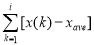(1)

1. We split the integrated data set into non-overlapping boxes of equal length, τ. In each box, a best polynomial local trend (of order n) is ï¬tted in order to detrend the integrated profile (by subtracting the locally fitted trend).
2. The root-mean-square fluctuations Fd (τ) of this integrated and detrended profile is calculated over all scales (box sizes). The detrended fluctuation function F is defined by: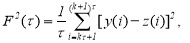k = 0, 1, 2,…,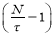, (2)

where z(i) is a linear least-square fit to the τ data contained within a box (Kantelhardt et al. 2002).

In case the signals involve scaling, a power-law behavior for the root-mean-square ï¬‚uctuation function Fd (τ) is observed:

Fd (τ) ∼ τa (3)

where α is the scaling exponent, a self-afï¬nity parameter that represents the long-range power-law correlation (Ausloos and Ivanova 2001).

It is worthy of note that a is a generalized Hurst exponent (H) being equal between them for the case of a fractional Gaussian noise (FGN) data set, where 0 < a < 1. For a fractional Brownian motion (FBM) data set (1 < a < 2) the relation between these exponents is H = a − 1 (Ausloos and Ivanova, 2001).

For uncorrelated data, the scaling exponent is α = 0.5. An exponent α ≠ 0.5 in a certain range of τ values implies the existence of long-range correlations in that time interval. If 0<α<0.5 (and if the data set is nonintermittent), power-law anticorrelations are present (antipersistence). When 0.5< α ≤1.0 (and if again the data set is nonintermittent), then persistent long-range power-law correlations prevail (the case α = 1 corresponds to the so-called 1/f noise) (Weber and Talkner 2001).

Finally, the scaling properties of SIF-WL data set were also studied using Haar analysis (Lovejoy and Schertzer, 2012a,b). According to Haar analysis the variation of X parameter fluctuations ΔX with scale Δt can be defined using the “generalized” qth order structure function Sqt) = < ΔΧt)q >, where the symbol <.> stands for ensemble averaging. In a scaling regime, Sqt) ≈ Δtξ(q), where the exponent ξ(q)= qH – K(q) and K(q) indicates the scaling intermittency (satisfying K(1) = 0).

3. Discussion and results

Varotsos et al. (2013a) studying the high-resolution observations of SIF reaching the ground and the top of the atmosphere, suggested that SIF versus ultraviolet WL exhibit 1/fï€­type power-law correlations. This result was derived by applying the DFA method on the SIF dataset obtained from the Villard St. Pancrace station of the Lille University of Sciences and Technology and was based on the slope (i.e., 1.02±0.02) of the log – log plot of the root mean square fluctuation function of SIF versus the WL segment size τ.

In order to further establish the power-law scaling mentioned before, Varotsos et al. (2013b) employed the two criteria suggested by Maraun et al. (2004), notably the rejection of the exponential decay of the autocorrelation function and the constancy of “local slopes” in a certain range towards the low frequencies. The results showed that the power-law fit on the power spectral density (of the detrended SIF-WL versus logτ) was much better than exponential one, for scales higher than τ ≈ 1.4 nm, while the method of local slopes exhibited “constancy” with constant threshold (a ≈ 0.92) at the same range, τ > 1.4 nm. Therefore, the two criteria of Maraum et al. (2004) were satisfied ensuring the 1/fï€­type power-law correlations in SIF versus WL suggested by Varotsos et al. (2013a).

In the present study, the scaling dynamics of a wider spectrum of SIF-WL data set was studied, for wavelengths between 115.5 and 629.5nm. Firstly, DFA-n seemed to can take care of the trends revealing a DFA-exponent close to unity (after DFA-2), as shown in Fig. 1b.

In the following we plotted the power spectral density (using FFT) of the linearly detrended SIF data set. The derived power spectral density showed that the power-law fitting gives coefficient of determination, R2, higher than the exponential one (see Fig. 2a). This was confirmed by F-test, at 95% confidence level. From the other hand, the DFA-1 exponent was 1.24, while by applying the multiple DFA on the linearly detrended SIF data, the derived exponents ranged from 0.94 to 1.

Similar results were derived when we repeated the above mentioned analysis for the polynomially (of 6th degree) detrended SIF data set. The derived power spectral density was, once more, better fitted algebraically than exponentially (a result which was confirmed by F-test, at 95% confidence level), while the linearly DFA-exponent was 1.01 (see Fig.2b). In other words, it seemed that no detrending is needed for the studied data set.

Next, to resume our results we analysed the initial SIF-WL data set by using Haar analysis (Lovejoy and Schertzer, 2012a,b). According to Haar analysis, as also mentioned in the Section 2, the variation of SIF fluctuations versus wavelength τ can be defined using the “generalized” qth order structure function Sq(τ) = < ΔRF(τ)q >, for which it holds that in a scaling regime Sq(τ) ≈ τξ(q), where the exponent ξ(q)= qH – K(q) and K(q) illustrates the scaling intermittency (satisfying K(1) = 0 and ξ(1) = H). Fig. 3b shows that the intermittency of SIF data set is very high (C1=0.20), hence the RMS exponent = ξ(2)/2=0.60 is quite different from the q=1 exponent (H) and the data are far from Gaussian. In the classical quasi-Gaussian case, K(q) = 0 so that ξ(q) is linear. More generally, if the field is intermittent – for example if it is the result of a multifractal process – then the exponent K(q) is generally non linear and convex and characterizes the intermittency. The physical significance of H is thus that it determines the rate at which mean fluctuations grow (H > 0) or decrease (H < 0) with scale τ. According to Fig. 3a,b, the exponent ξ(2) of the structure function equals to zero (at scales below 10 nm), a fact which means that the power spectrum exponent β=1+ξ(2) equals to 1 (1/f structure). On the other hand, at larger scales, the exponents ξ(2) and β seem to equal to 1.2 and 2.2, respectively.

To clarify this aspect, we calculated the power spectrum for the initial SIF-WL data set, using the MEM. Then, we plotted in Fig. 4a the power spectral density versus frequency and we compared these results with 1/f and 1/f2.2 structure, following the exponent ξ(2)= 1.2 shown in Fig. 3. Interestingly, the results lied in between these two lines.

Furthermore, we calculated the power spectrum for the initial as well as for the polynomially (of 6th and 7th degree) detrended SIF data set, using again the MEM. We plotted in Fig. 4b the power spectral density versus WL and the corresponding algebraically fitting in the range from 10 to 100 nm. In the latter fitting we found exponent very close to unity.

However, Varotsos et al. (2013a) tried to formulate the above-shown finding, i.e., that the solar spectral irradiance obeys 1/f power-law as a function of UV wavelength, using the well-known Planck’s law: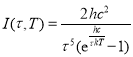which, in the limit of small wavelengths tends to the Wien approximation: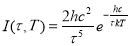, where I(τ,Τ) is the amount of energy emitted at a wavelength τ per unit surface area per unit time per unit solid angle per unit wavelength, T is the temperature of the black body, h is Planck’s constant, c is the speed of light, and k is Boltzmann’s constant. By applying the DFA method on the various values of I(τ,Τ) Varotsos et al. (2013a) showed that the calculated I(τ,Τ) values do not obey the 1/fï€­type scaling.

In an effort to clarify this aspect, we firstly applied on the initial SIF data set a fitting that was based on the Planck formula: Planck(τ)=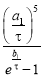and the derived exponents were a1 = 6799.86± 45.39(0.6674%) and b1= 2754.38 ± 15.85 (0.5755%) (see Fig. 5). Next, we applied the formula: p(τ)=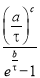which releases the exponent in front of the Bose-Einstein distribution giving a possible explanation for the different distribution of photons. The derived exponents were a= 3250.17 ± 90.65 (2.79%), b= 4456.26 ± 121.3 (2.72%) and c= 8.81914 ± 0.2589 (2.94%). Our last step was to use the Planck formula taking into account the effective temperature obtained from NASA.

However, since hc = 1.986ïƒ-10-25 Jm and k = 1.38ïƒ-10-23 J/K, the Planck formula gives Τsun = 5224.3 K while the p(τ) formula gives Τsun = 3229.5 K. Furthermore, the Wien displacement law: τmax ïƒ-T = b, where τmax = 450 nm is the peak wavelength and b = 2.898ïƒ-10−3mK is a constant of proportionality called Wien’s displacement constant, gives Τsun = 6440K.

The analysis based on Planck and Wien laws gives Τsun = (5224 + 6440) / 2 K = 5832 K which is less than 1% from the effective temperature obtained from NASA.

Finally, the application of DFA method on the detrended SIF data, eliminating the fitting of Planck, p(τ) and Plank(τ, Tef) formula, gave again DFA-exponents very close to 1 (ranging from 1.01 to 1.08) as shown in Fig. 6.

All the previous discussion indicates that the 1/f scaling dynamics observed in solar flux concerns not the Planck’s law but its variations.

4. Conclusions

The main conclusions of the present survey were:

1. DFA-n applied on the initial SIF data set revealed DFA-exponents very close to unity (after DFA-2).
2. Power spectral density for the linearly detrended SIF data set showed that the power-law fitting gives coefficient of determination, R2, higher than the exponential one, while DFA-1 exponent was 1.24 and DFA-n exponents ranged from 0.94 to 1. Similar results were extracted when we repeated the above mentioned analysis for the polynomially (of 6th degree) detrended SIF data set and it seemed that no detrending is needed for the studied data set.
3. To resume our results we analysed the initial SIF-WL data set by using Haar analysis. As ιt was derived, the intermittency of SIF data set was very high and the data were far from Gaussian. At scales below 10 nm, the power spectrum exponent β was almost 1 (1/f structure), while at larger scales, the exponents ξ(2) and β seemed to equal to 1.2 and 2.2, respectively.
4. The results of the power spectral density for the initial SIF-WL data set (using the MEM) versus frequency seemed to lie in between 1/f and 1/f2.2 structure.
5. The power spectral density versus WL for the initial as well as for the polynomially (of 6th and 7th degree) detrended SIF data set (using again the MEM) revealed also exponent very close to unity.
6. Eliminating the fitting of three different types of Planck formula from SIF data set, scaling exponents very close to unity were derived

Finally, the 1/f scaling dynamics observed in solar flux concerns not the Planck’s law but its variations.

## Related Services

View all

### DMCA / Removal Request

If you are the original writer of this essay and no longer wish to have your work published on the UKDiss.com website then please:

Related Services